Slopes of Parallel and Perpendicular LinesCustom SearchSLOPES OF PARALLEL AND PERPENDICULAR LINES If two lines are parallel, their slopes must be equal. Each line will cut the X axis at the same angle a so that if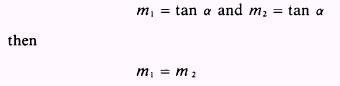We conclude that two lines which are parallel have the same slope. Suppose that two lines are perpendicular to each other, as lines L1 and L2 in figure 1-5. The slope and angle of inclination of L1 are m1 and a1, respectively. The slope and angle of inclination of L2 are m2 and a2, respectively. Then the following is true: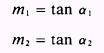Although not shown here, the fact that a2 (fig�1-5) is equal to a, plus 90 � can be proven geometri�cally. Because of this relationship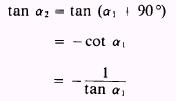Figure 1-5.-Slopes of perpendicular lines.   Replacing tan a, and tan aZ by their equivalents in terms of slope, we haveWe conclude that if two lines are perpendicular, the slope of one is the negative reciprocal of the slope of the other. Conversely, if the slopes of two lines are negative reciprocals of each other, the lines are perpendicular.       EXAMPLE: In figure 1-6 show that line L1 is perpendicular to line L2. Line L1 passes through points P1(0,5) and P2 (-1,3). Line L2 passes through points P2(-1,3) and P3(3,1).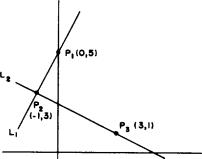Figure 1-6.-Proving lines perpendicular. SOL UTION: Let m1 and M2 represent the slope of lines L1 and L2, respectively. Then we have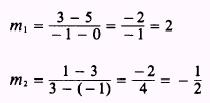Since their slopes are negative reciprocals of each other, the lines are perpendicular. PRACTICE PROBLEMS: 1. Find the distance between P1 (5,3) and P2 (6,7). 2. Find the distance between P1 (1/2,1) and P2 (3/2,5/3). 3. Find the midpoint of the line connecting P1 (5,2) and P2 (-1, -3). 4. Find the slope of the line joining P1 (-2,- 5) and P2 (2,5). 5. Find the slope of the line perpendicular to the line joining P1(-3,6) and P2(-5,-2). ANSWERS: 1, 17Integrated Publishing, Inc. - A (SDVOSB) Service Disabled Veteran Owned Small Business